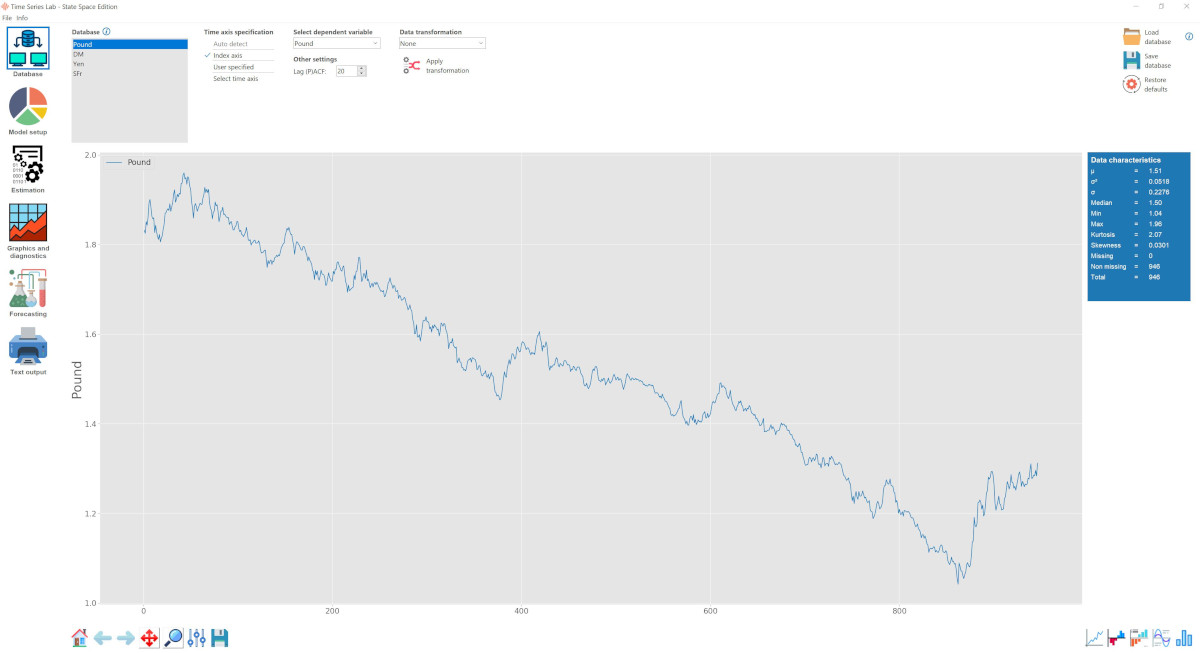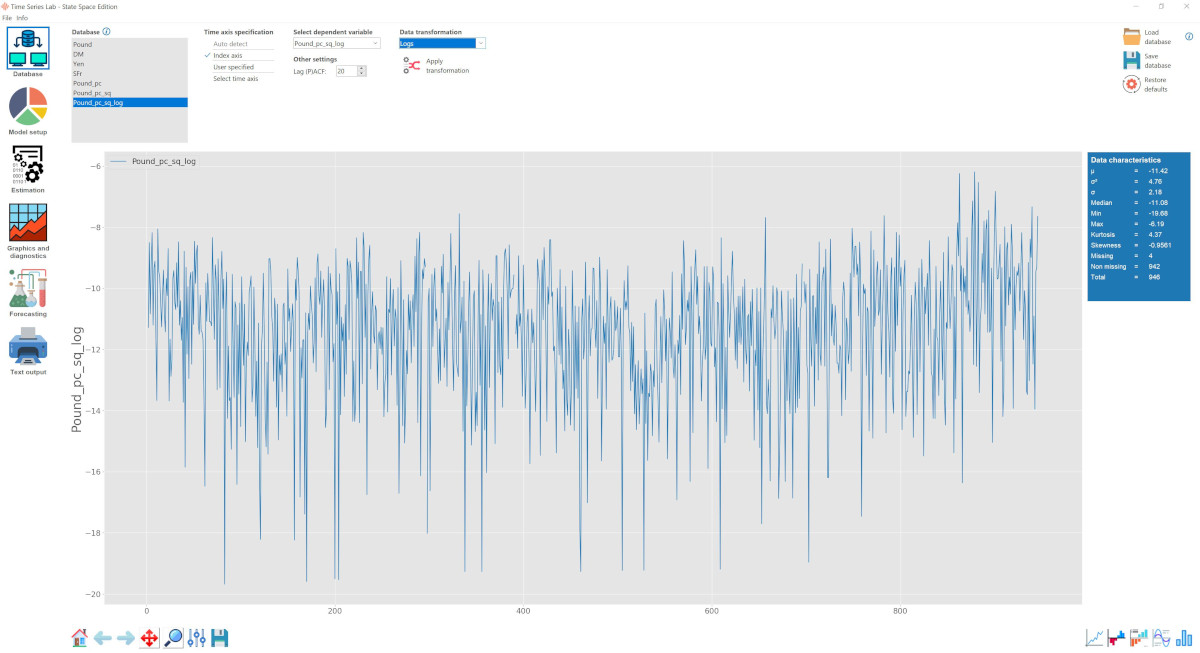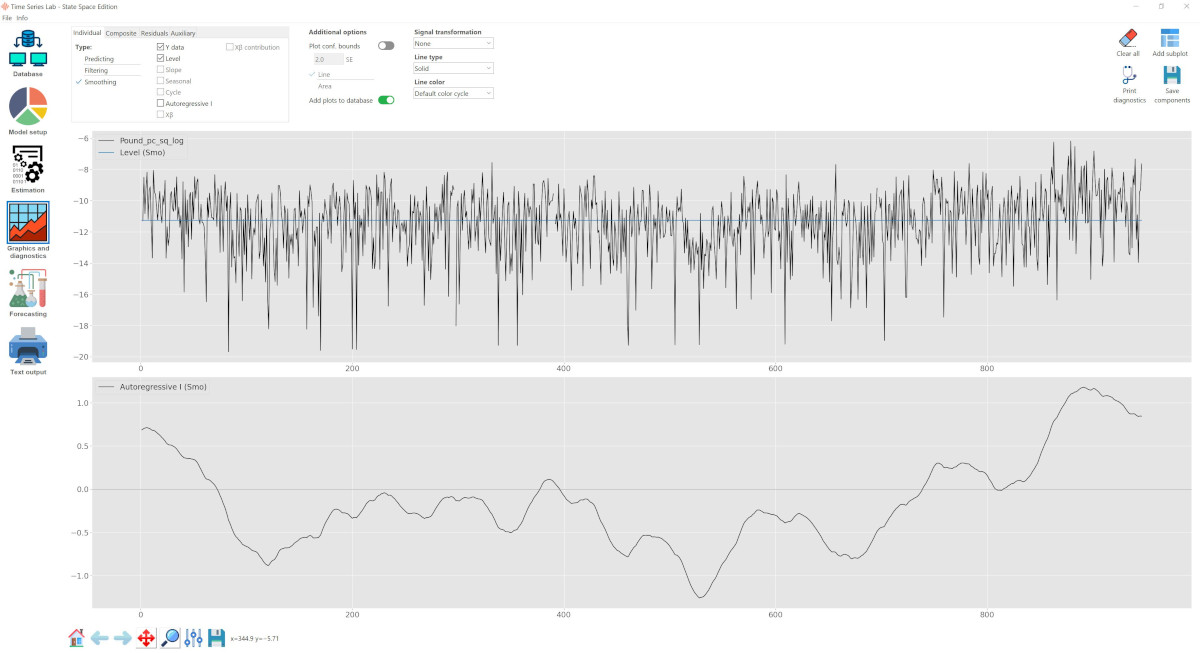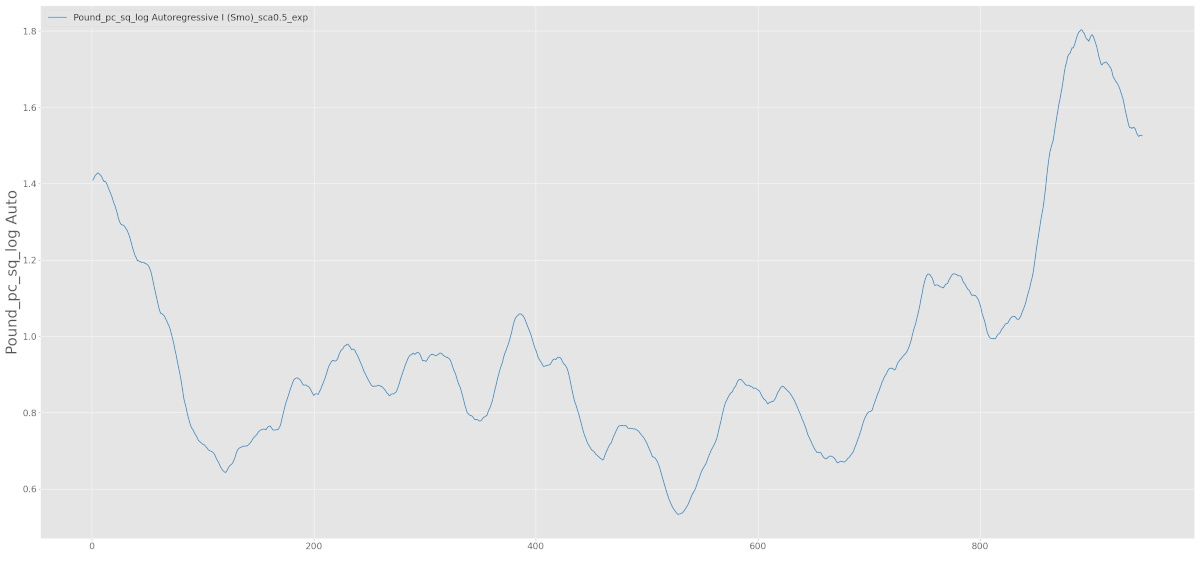# Case Studies

The Time Series Lab (TSL) software packages make time series analysis available to anyone with a basic knowledge of statistics. The program is written in such a way that results can be obtained quickly. However, many advanced options are available for the time series experts among us. The modelling process in TSL consists of a five step procedure: Database, Model setup, Estimation, Graphics & diagnostics, and Forecasting. In our case studies, we often present screenshots of the program so that you can easily replicate results.Did you know you can make a screenshot of a TSL program window? Press Ctrl + p to open a window which allows you to save a screenshot of the program. The TSL window should be located on your main monitor.

Click on the buttons below to go to our case studies. At the beginning of each case study, the required TSL package is mentioned. Our first case study, about the Nile data, is meant to illustrate the basic workings of the program.

# Stochastic Volatility

Rutger Lit
September 25, 2021
TSL module: State Space Edition v1.01 - Univariate Basic
Topics: variable transformation, autoregressive process

Let $y_t$ be a stock series returns or the difference of logged exchange rates. Such a series will normally be approximately white noise. However it may not be independent because of serial dependence in the variance. This can be modelled by \begin{equation}\label{sv1} y_t = \sigma_t \epsilon_t = \sigma \epsilon_t \text{exp}(h_t/2), \quad \epsilon_t\sim \text{IID}(0, 1), \quad t = 1,\ldots,T \end{equation} where \begin{equation}\label{sv2} h_{t+1} = \phi h_t + \eta_t, \quad \eta_t \sim \text{NID}(0,\sigma^2_{\eta}), \quad |\phi| \leq 1. \end{equation} The term $\sigma^2$ is a scale factor, $\phi$ is a persistence parameter, and $\eta_t$ is a disturbance term which in the simplest model is uncorrelated with $\epsilon_t$. Literature reviews are given by Shephard (2005) and Ghysels, Harvey, and Renault (1996). This stochastic volatility (SV) model has two main attractions. The first is that it is the natural (Euler) discrete time analogue of the continuous time model used in papers on option pricing, such as Hull and White (1987). The second is that its statistical properties are easy to determine. The disadvantage with respect to the conditional variance models of the GARCH class is that likelihood based estimation can only be carried out by a computer intensive technique such as that described in Kim, Shephard, and Chib (1998) and Sandmann and Koopman (1998). However, a quasi-maximum likelihood (QML) method is relatively easy to apply and is often reasonably efficient. This method is based on transforming the observations to give: \begin{equation}\label{sv3} \text{log}\, y^2_t = \kappa + h_t + \xi_t, \quad t = 1,\ldots,T \end{equation} where \begin{equation} \xi_t = \text{log}\, \epsilon^2_t - E(\text{log}\, \epsilon^2_t) \end{equation} and \begin{equation} \kappa = \text{log}\, \sigma^2 + E(\text{log}\, \epsilon^2_t) \end{equation} As shown in Harvey, Ruiz, and Shephard (1994), the state space form given by equations \eqref{sv1} and \eqref{sv2} provides the basis for QML estimation via the Kalman filter and also enables smoothed estimates of the variance component, $h_t$, to be constructed and predictions made. One of the attractions of the QML approach is that it can be applied without the assumption of a particular distribution for $\epsilon_t$. In Harvey, Ruiz, and Shephard (1994), the volatility in the daily exchange rate of the US dollar against four currencies is examined; see also Mahieu and Schotman (1998).

The data are in the file EXCH.csv which is part of any TSL - State Space Edition installer file with a version number higher than v1.00 and can be found in the data folder located in the install folder of TSL - State Space Edition. The file EXCH.csv consists of daily exchange rates for the US dollar. The four series are the daily exchange rates of the dollar against the pound, deutschmark, yen and Swiss franc. The data were recorded at the end of each weekday from 1/10/81 until 28/6/85. Thus the sample size is 946.

Step 1 is to load the time series data and transform it so that we can model the data as in equation \eqref{sv3}.

In the figure below, the EXCH dataset is loaded into TSL and the Pound / Dollar exchange rate is highlighted.Time Series Lab - State Space Edition Database page. The Pound / USD exchange rate is highlighted.

Our next task is to obtain returns from the exchange rates. We do this by taking first differences of the logarithm of the exchange rates.

Highlight the Pound series ► Select Percentage change from the Data transformation pull-down menu ► Click on the Apply transformation button.

A new variable should appear in the Database section with the name Pound_pc. This new variable corresponds with the $y_t$ variable in equation \eqref{sv3}. Next we need to square the series and finally take logs to equal the left hand side of equation \eqref{sv3}.

Highlight the Pound_pc series ► Select Square from the Data transformation pull-down menu ► Click on the Apply transformation button. ► Select Logs from the Data transformation pull-down menu ► Click on the Apply transformation button.
We should now see the figure below.Time Series Lab - State Space Edition Database page. The transformed Pound / USD exchange rate series is highlighted.

Subsequently we fit an AR(1) + Irregular + Fixed Level model. Go to
Model setup page ► Select a fixed Level (no slope) ► select an Autoregressive process of order 1.
Go to Estimate page ► Estimate.
We should now see the Figure below. The smoothed estimate of the volatility process, $h_t$, is displayed in the usual way by selecting the Autoregressive I process on the Graphics and Diagnostics page. If we select the Exponential option in the Signal transformation pull-down menu we can plot the exponent of the smoothed volatility. This may be interpreted as the ratio of the volatility to the underlying level.Time Series Lab - State Space Edition graphics page with (fixed) Level and Autoregressive process plotted.

It may be preferable to consider the variations in the standard deviation, $\text{exp}(h_t / 2)$, in which case we go back to the Database page ► select the Pound_pc_sq_log Autoregressive I (Smo) series ► Scale the series by 0.5 ► take the exponent of the series. This should lead to the last Figure below.Estimated $\text{exp}(h_t / 2)$ for the Pound series.

# Bibliography

### References

Shephard, N., 2005. Stochastic Volatility: Selected Readings. Oxford University Press, Oxford.

Ghysels, E., A. C. Harvey, and E. Renault (1996). Stochastic volatility. In C. R. Rao and G. S. Maddala (Eds.), Statistical Methods in Finance, pp. 119–91. Amsterdam: North-Holland.

Hull, J. and A. White (1987). The pricing of options on assets with stochastic volatilities. J. Finance 42, 281–300.

Kim, S., N. Shephard, and S. Chib (1998). Stochastic volatility: likelihood inference and comparison with ARCH models. Rev. Economic Studies 65, 361–93.

Sandmann, G. and S. J. Koopman (1998). Estimation of stochastic volatility models via Monte Carlo maximum likelihood. J. Econometrics 87, 271–301.

Harvey, A. C., E. Ruiz, and N. Shephard (1994). Multivariate stochastic variance models. Rev. Economic Studies 61, 247–64.

Mahieu, R. and P. Schotman (1998). An empirical application of stochastic volatility models. J. Applied Econometrics 16, 333–59.